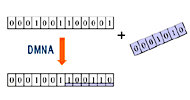## The main components of DMNA

The revolutionary algorithm DMNA utilizes mathematical solutions such as factorization, fold operations, and hierarchical processing to drastically reduce redundant calculations in algorithms such as DCT (Discrete Cosine Transform) and ME (Motion Estimation).
For example, the DCT and inverse DCT operations that are often used in image and video compression processing such as the above equation.
This complex operation can be converted into a simple combination of calculations by using DMNA to achieve a significant speed-up operation.

### Dragon1: Factorization

 Factorization is used to simplify the calculations. The number of calculations has been significantly reduced.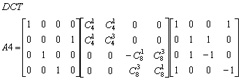### Dragon2: Fold back

 By Utilizing the fold theory, we can narrow the numerical space and reduce the number of operations.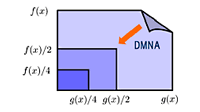### Dragon3: Hierarchy of operations (1)

 Hierarchize calculation formulas and prioritization enable to perform only the necessary calculations.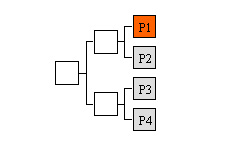### Dragon3: Hierarchy of operations (2)

 Layered complicated calculation simplify the hierarchical calculations.### Dragon4: Function Conversion

 Each function can be efficiently converted.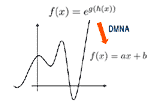### Dragon5: Encryption

 Enable to embed the highly encrypted data without increasing the amount of data.